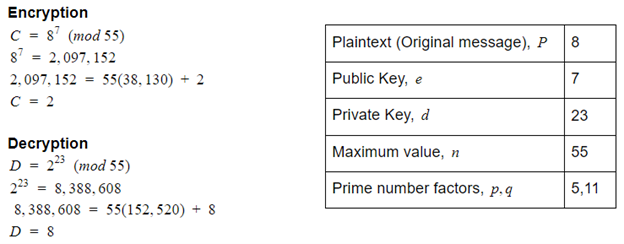Search

# Cryptography: keeping information secret

By Gemma Penson - Computer Science Student @ Trinity Hall, Cambridge

Cryptography is the study of techniques that can be used to keep data secure when it’s being transmitted, so that only the sender and intended recipient of a message can view its contents. This is done as otherwise someone may be able to intercept your bank details during an online payment and wreak all kinds of unwanted criminal havoc. A system that uses cryptography is known as a cryptosystem, with cryptosystems often using three algorithms: one for key generation, one for encryption, and one for decryption.

So what is a key? It’s a string of characters, often numbers, that is used for altering data such as a text message. For example, some keys alter data so that it appears random which disguises the contents of the data when it’s transmitted across a network. This type of key is known as a public key and the process is known as encryption. Encryption is similar to locking your house door in the physical world and it’s used to convert readable plaintext, like your text message, into unreadable, random-looking ciphertext.

The inverse of encryption is decryption (unlocking), which converts the ciphertext back into plaintext so that your recipient can read the message that you sent. The public and the private key used are mathematically linked so that the decryption process yields the expected plaintext and not more random-looking data.

In a public key cryptosystem, each user has their own public and private key, with it being near to impossible to compute someone’s private key if you have their public key. Just as the names suggest, each user’s public key can be widely distributed,whereas their private key should only be known by the user.

These keys are far too long for you to remember so they’re stored digitally. Your public key is stored in a document known as a digital certificate, which is looked after by a certificate authority and is used to prove that you’re the owner of that particular key. On the other hand, your private key is stored in your computer’s operating system where it’s much less likely to be found than if it was stored online.

The classic example of the public key cryptosystem is when Alice wants to send a message to Bob. To send the message securely, Bob sends his public key to Alice over the communication network which Alice uses to encrypt her message. Alice then sends her disguised ciphertext over the network, which can’t be read by snooping Eve who may intersect the message on its journey to Bob.

When Bob receives the ciphertext, he has to decrypt it before he’s able to read Alice’s message, which is done using his private key.There are many different types of public key cryptography systems which each use different key formats and algorithms in order to encrypt and decrypt your data. One of the most well-known systems is RSA, which was introduced in 1977. It uses numeric keys and its security is based on the theory of numbers as it harnesses the idea that factorisation takes longer than multiplication to complete.

Using large numbers without a constraint wouldn’t be efficient so first a maximum value is obtained by multiplying two randomly generated prime numbers. The public key is then mathematically chosen in a way that ensures that it falls in the range 0 < x < max.

As long as the maximum value and its factors are known, the private key can then be computed from the public key using the Extended Euclidean Algorithm. This algorithm ensures that the private key generated can be used to successfully decrypt a message that was encrypted using the public key.

Encrypting a number can be done by multiplying the number by itself e times, where e is the public key. Numbers are treated like the numbers on a clock: if the calculation results in a number greater than the maximum value n, the output should be wrapped so that it is within the range 0 < x < n.

In RSA, this wrapping is achieved by dividing the value obtained from the calculation above by the maximum value and taking the remainder as the new value. This is known as finding the remainder modulo of n.

To decrypt the number, it is multiplied by itself d times, where d is the private key. The remainder modulo of n is then found, which will equal the initial number that we started with.

Here is a mathematical summary of these operations:The public and private keys (the exponents e and d) are chosen in a way that D = P: the message that has been encrypted and then decrypted is the same as the initial message. The effect of the two operations is to reproduce the initial number:

Where the remainder modulo of n can just be found once instead of twice.

Here is a worked example of RSA:If you were able to factorise the maximum value of 55 into its component primes you would be able to compute the private key from the public key. This would mean that sneaky Eve could decrypt the incoming message to see what Alice was sending Bob, causing a security breach.

Whilst multiplying the prime numbers together is relatively straightforward, factorising the maximum value into its prime components is considered to be mathematically tricky, especially when large prime numbers are used. When RSA was first introduced, there wasn’t a more optimal factorisation method than using a brute-force approach. However, in recent years, algorithms have been introduced that are able to factorise numbers much more efficiently and thus RSA has become less secure over time.